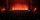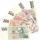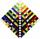# Apples in baskets

Determine how many apples are in baskets when in the first basket are 4 apples, and in any other is 29 apples more than the previous, and we have eight baskets.

Result

s8 =  207

#### Solution:Leave us a comment of example and its solution (i.e. if it is still somewhat unclear...):Be the first to comment!## Next similar examples:

1. StudentsIn the front row sitting three students and in every other row 11 students more than the previous row. Determine how many students are in the room when the room is 9 lines, and determine how many students are in the seventh row.
2. Consecutive numbersSum of ten consecutive numbers is 105. Determine these numbers (write first and last).
3. AP - simpleDetermine the first nine elements of sequence if a10 = -1 and d = 4
4. AS sequenceIn an arithmetic sequence is given the difference d = -3 and a71 = 455. a) Determine the value of a62 b) Determine the sum of 71 members.
5. SeatsSeats in the sport hall are organized so that each subsequent row has five more seats. First has 10 seats. How many seats are: a) in the eighth row b) in the eighteenth row
6. Saving per centsThe first day I save 1 cent and every next day cent more. How many I saved per year (365 days)?
7. TreesA certain species of tree grows an average of 0.5 cm per week. Write an equation for the sequence that represents the weekly height of this tree in centimeters if the measurements begin when the tree is 200 centimeters tall.
8. SequenceWrite the first 6 members of these sequence: a1 = 5 a2 = 7 an+2 = an+1 +2 an
9. Sequence 2Write the first 5 members of an arithmetic sequence a11=-14, d=-1
10. SequenceWrite the first 7 members of an arithmetic sequence: a1=-3, d=6.
11. 6 termsFind the first six terms of the sequence. a1 = 7, an = an-1 + 6
12. Sum of membersWhat is the sum of the first two members of the aritmetic progression if d = -4.3 and a3 = 7.5?
13. Sequence 3Write the first 5 members of an arithmetic sequence: a4=-35, a11=-105.
14. SequenceBetween numbers 1 and 53 insert n members of the arithmetic sequence that its sum is 702.
15. Theorem proveWe want to prove the sentence: If the natural number n is divisible by six, then n is divisible by three. From what assumption we started?
16. SeriesYour task is express the sum of the following arithmetic series for n = 14: S(n) = 11 + 13 + 15 + 17 + ... + 2n+9 + 2n+11
17. Nineteenth memberFind the nineteenth member of the arithmetic sequence: a1=33 d=5 find a19